output.to from Sideway
Mechanics: Statics

3D Body

Draft for Information Only

# Content

``` Theorems of Pappus-Guldinus   Theorem 1: Surface of Revolution   Surface of Revolution  Theorem 2: Body of Revolution   Body of Revolution  Applications of Theorems of Pappus-Guldinus   Surface of Revolution   Solid of Revolution```

# Theorems of Pappus-Guldinus

The theorems of Pappus-Guldinus were formulated by the Greek geometer Pappus of Alexandria during the 4th century A.D. (about 340 A.D.) and were restated by the Swiss mathematician Paul Guldinus (1640). The two theorems of Pappus-Guldinus describe the area of surface of revolution and the volume of body of revolution by the circular path traversed by their centroid during the revolution.

## Theorem 1: Surface of Revolution

For the surface of a surface of revolution generated by the rotation of a plane curve about a non-intersecting axis, the surface area A of the surface of revolution is equal to the product of the curve length L of the generating curve and the travelled distance d of the centroid of the generating curve during the generation of the surface by revolution. And the travelled distance of the centroid by revolution can also be expressed in terms of the perpendicular distance y of the centroid away from the rotating axis.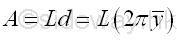### Surface of RevolutionThe area A of the surface of revolution can be determined by integration through the revolution of an elemental segment dL. ImplyThe area A can be rearranged in the form of the integral of the first moment of an elemental segment , which can also be expressed in terms of the centrod of the generating curve, Imply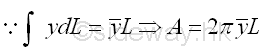## Theorem 2: Body of Revolution

For the body of a body of revolution generated by the rotation of a plane region about a non-intersecting axis, the body volume V of the body of revolution is equal to the product of the area A of the generating plane region and the travelled distance d of the centroid of the generating region during the generation of the body by revolution. And the travelled distance of the centroid by revolution can also be expressed in terms of the perpendicular distance y of the centroid away from the rotating axis.### Body of Revolution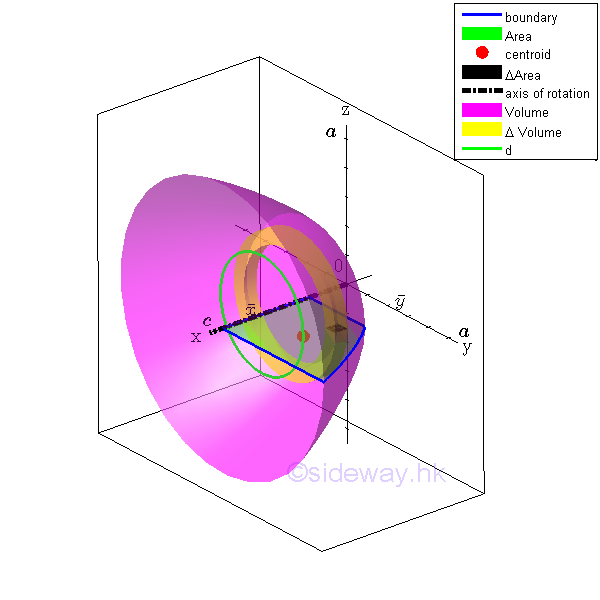The volume V of the body of revolution can be determined by integration through the revolution of an elemental area dA. Imply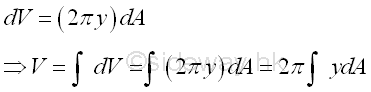The volume V can be rearranged in the form of the integral of the first moment of an elemental area, which can also be expressed in terms of the centrod of the generating area, Imply## Applications of Theorems of Pappus-Guldinus

The Theorems of Pappus-Guldinus provides a simple relationship between the area of surface of revolution or the volume of body of revolution and the centroid of the generating plane curve or the centroid of the generating plane area. Therefore the Theorems of Pappus-Guldinus can be used to determine the area of surface of revolution and the volume of body of revolution from the generating curve and the generating area accordingly. And the centroid of a generating plane curve and the centroid of a generating plane area can also be determined from the surface of revolution and body of revolution accordingly.

### Surface of Revolution

CylinderArea of cylinder of surface of revolution is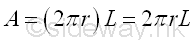Area of cylinder of surface of revolution by theorem of Pappus-Guldinus is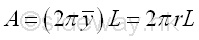Cone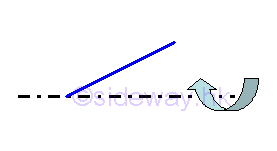Area of cone of surface of revolution is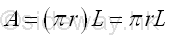Area of cone of surface of revolution by theorem of Pappus-Guldinus isSphere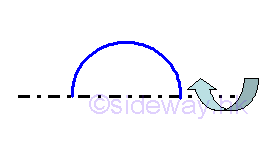Area of sphere of surface of revolution isArea of sphere of surface of revolution by theorem of Pappus-Guldinus is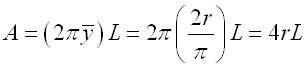Torus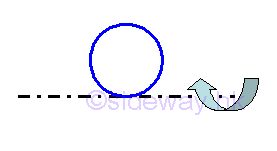Area of torus of surface of revolution is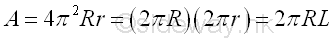Area of torus of surface of revolution by theorem of Pappus-Guldinus is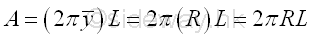### Solid of Revolution

Cylinder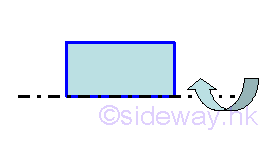Volume of cylinder of body of revolution is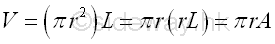Volume of cylinder of body of revolution by theorem of Pappus-Guldinus isCone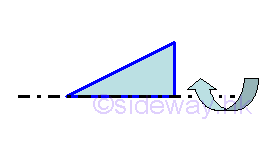Volume of cone of surface of revolution is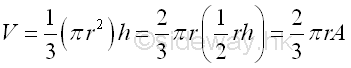Volume of cone of surface of revolution by theorem of Pappus-Guldinus is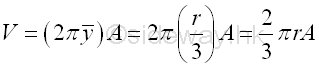SphereVolume of sphere of surface of revolution is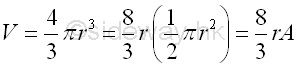Volume of sphere of surface of revolution by theorem of Pappus-Guldinus is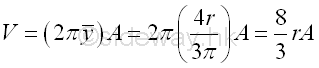Torus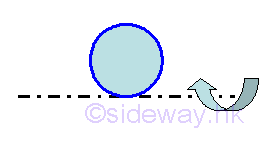Volume of torus of surface of revolution isVolume of torus of surface of revolution by theorem of Pappus-Guldinus is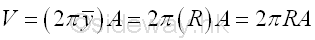©sidewayReferences

1. I.C. Jong; B.G. rogers, 1991, Engineering Mechanics: Statics and Dynamics, Saunders College Publishing, United States of America
2. F.P. Beer; E.R. Johnston,Jr.; E.R. Eisenberg, 2004, Vector Mechanics for Engineers: Statics, McGraw-Hill Companies, Inc., New YorkID: 120700003 Last Updated: 2012/7/9 Revision: 0 Ref:Home (5)

Business

Management

HBR (3)

Information

Recreation

Hobbies (7)

Culture

Chinese (1097)

English (336)

Reference (66)

Computer

Hardware (149)

Software

Application (187)

Digitization (24)

Numeric (19)

Programming

Web (648)CSS (SC)

ASP.NET (SC)

HTML

Knowledge Base

Common Color (SC)

Html 401 Special (SC)

OS (389)

MS Windows

Windows10 (SC)

.NET Framework (SC)

DeskTop (7)

Knowledge

Mathematics

Formulas (8)

Number Theory (206)

Algebra (20)

Trigonometry (18)

Geometry (18)

Calculus (67)

Complex Analysis (21)

Engineering

Tables (8)

Mechanical

Mechanics (1)

Rigid Bodies

Statics (92)

Dynamics (37)

Fluid (5)

Control

Acoustics (19)

Biology (1)

Geography (1)

Copyright © 2000-2019 Sideway . All rights reserved Disclaimers last modified on 10 Feb 2019